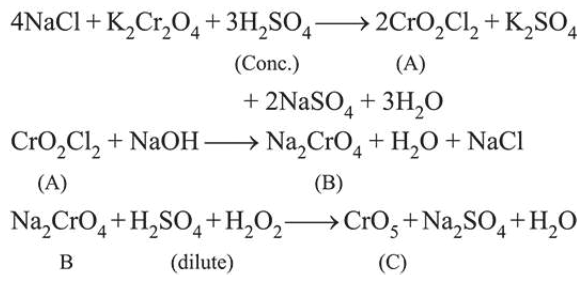# Consider the following reactions:Question:

Consider the following reactions:

$\mathrm{NaCl}+\mathrm{K}_{2} \mathrm{Cr}_{2} \mathrm{O}_{7}+\mathrm{H}_{2} \mathrm{SO}_{4} \rightarrow(\mathrm{A})+$ Side products (Conc.)

$(A)+\mathrm{NaOH} \rightarrow(\mathrm{B})+$ Side products

(B) $+\mathrm{H}_{2} \mathrm{SO}_{4}+\mathrm{H}_{2} \mathrm{O}_{2} \rightarrow$ (C) $+$ Side products (dilute)

The sum of the total number of atoms in one molecule each of $(A),(B)$ and $(C)$ is ______________ .

Solution:

(18.00)